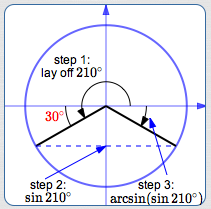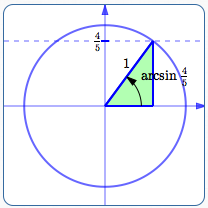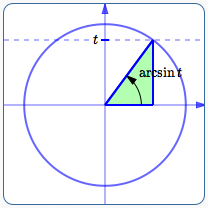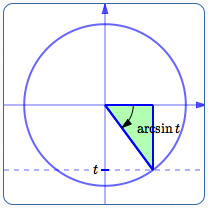﻿ Inverse Trigonometric Function Problems: All Mixed Up

# Inverse Trigonometric Function Problems: All Mixed Up

• PRACTICE (online exercises and printable worksheets)

This section gives mixed practice with the three most common inverse trigonometric functions:

All problems should be done without a calculator.

## Example 1

Compute:   $\,\arcsin ( \sin 210^\circ)$

Solution:
Caution! Since the sine and arcsine functions are not true inverses, they do not necessarily ‘undo’ each other.
Indeed, $\,\arcsin( \sin 210^\circ)\,$ cannot equal $\,210^\circ\,$, since the arcsine always returns an angle between $\,-90^\circ\,$ and $\,90^\circ\,$.

Work from the ‘inside’ of the expression to the ‘outside’: $$\overset{\text{step 3}} {\overbrace{\arcsin (\ \underset{\text{step 2}} { \underbrace{\sin \overset{\text{step 1}} {\overbrace{210^\circ}} }}\ )}}$$

 Step 1: As shown at right, lay off a $\,210^\circ\,$ angle ($\,210^\circ = 180^\circ + 30^\circ\,$) in the unit circle. Recall that positive angles are laid off in a counterclockwise direction. Observe that the reference angle for $\,210^\circ\,$ is $\,\color{red}{30^\circ}\,$.Step 2: By definition of the sine function, $\,\sin 210^\circ\,$ is the $y$-value of the terminal point. Step 3: Apply the arcsine function: What angle between $\,-90^\circ\,$ and $\,90^\circ\,$ has this $y$-value? Answer: $\,-30^\circ$ Report the answer: $\arcsin(\sin 210^\circ) = -30^\circ$

## Example 2

Find:   $\,\tan(\arcsin \frac 45)\,$

 Step 1: Show the angle   $\,\arcsin \frac{4}{5}\,$   on a unit circle. It is the unique angle between $\,-90^\circ\,$ and $\,90^\circ\,$ whose sine value is $\,\frac 45\,$; i.e., the unique angle (in the first quadrant) whose terminal point has $y$-value equal to $\,\frac 45\,$.Step 2: Take the green triangle (which has hypotenuse of length $\,1\,$ and vertical side of length $\,\frac 45\,$) and multiply all the side lengths by $\,5\,$. This gives a similar triangle with side lengths that are much easier to work with! Alternate approach for steps 1 and 2: use the right triangle approach only: sine is opposite over hypotenuse draw a right triangle with an acute angle labeled $\,\arcsin \frac 45\,$ mark lengths on the opposite side and hypotenuse, using: $\,\text{sine} = \frac 45 = \frac{\text{OPP}}{\text{HYP}}$Step 3: Compute the remaining side length in the triangle: immediately recognize the special $\,\color{red}{3}{-}4{-}5\,$ right triangle or use the Pythagorean theorem: $\,x^2 + 4^2 = 5^2\,$,   so $\,x = \color{red}{3}\,$ Step 4: Tangent is opposite over adjacent:   $\displaystyle\tan(\arcsin \frac 45) = \frac{\text{OPP}}{\text{ADJ}} = \frac 43$

## Example 3

Rewrite as an algebraic expression in $\,t\,$:   $\,\tan(\arcsin t)\,$

This is similar to the prior example, except for the variable $\,t\,$ instead of the constant $\,\frac 45\,$.
For what values of $\,t\,$ is $\,\arcsin t\,$ defined?   In other words, what is the domain of the arcsine function?   Answer:   $\,t\in[-1,1]\,$

Initially, we'll suppose that $\,0 < t < 1\,$, so you can see the similarity to the prior example.
Then, we'll see that the formula obtained also works for $\,t = 0\,$ and $\,-1 < t < 0\,$.

 Step 1: Let $\,0 < t < 1\,$. Show the angle   $\,\arcsin t\,$   on a unit circle. It is the unique angle between $\,-90^\circ\,$ and $\,90^\circ\,$ whose sine value is $\,t\,$; i.e., the unique angle (in the first quadrant) whose terminal point has $y$-value equal to $\,t\,$.Step 2: Or, use the right triangle approach: sine is opposite over hypotenuse, so $$\text{sine} = t = \frac {t}{1} = \frac{\text{OPP}}{\text{HYP}}$$ Mark the opposite side as $\,t\,$ and the hypotenuse as $\,1\,$.Step 3: Compute the remaining side length in the triangle: $$\begin{gather} x^2 + t^2 = 1^2\cr x^2 = 1 - t^2\cr x = \pm\sqrt{1 - t^2} \end{gather}$$ Since $\,x > 0\,$ in this initial discussion, choose the ‘$+$’ sign. Step 4: Tangent is opposite over adjacent:   $\displaystyle\tan(\arcsin t) = \frac{\text{OPP}}{\text{ADJ}} = \frac{t}{\sqrt{1-t^2}}$ Step 5: Note that the formula also works for $\,t = 0\,$, as follows: $\tan (\arcsin 0) = \tan 0 = 0$ for $\,t = 0\,$, $\frac{t}{\sqrt{1-t^2}} = \frac{0}{\sqrt{1 - 0^2}} = 0$ Step 6: Note that the formula also works for $\,-1 < t < 0\,$, as follows: In this case, $\,\arcsin t\,$ is in Quadrant IV. In Quadrant IV, the tangent is negative. The size of $\,\tan (\arcsin t)\,$ is the same as in Quadrant I. So, we want a formula that has the same size as $\,\frac{t}{\sqrt{1-t^2}}\,$ and is negative. Note that when $\,t\,$ is negative, so is $\,\frac{t}{\sqrt{1 - t^2}}\,$ (since it has a negative numerator and a positive denominator). Together, $\,\tan (\arcsin t) = \frac{t}{\sqrt{1-t^2}}\,$ for $\,-1 < t < 0\,$.Step 7: Combining all the cases: $$\tan (\arcsin t) = \frac{t}{\sqrt{1 - t^2}}\ \ \ \text{for}\ \ \ -1 \le t\le 1$$

## If you only need a reliable answer ...

Zip up to WolframAlpha and type in:

arcsin ( sin(210 deg) )

tan( arcsin (4/5) )

tan( arcsin t )

Master the ideas from this section